## What is Return on Investment ROI?

The Return on Investment metric compares investment gains directly to investment costs. Simple ROI, therefore, is rightly said to measure profitability.

Do investment returns justify investment costs? Does the investment return acceptable profits? Exactly how profitable is the investment? Return on Investment ROI metrics are designed to address such questions.

Investors frequently apply the metric to traditional financial investments, such as bonds, stock shares, certificates of deposit, or the use of venture capital. However, others use the same metric to support decisions about a wide range of actions or decisions they view essentially as business investments. Proposal review boards, for instance, routinely ask for an ROI with incoming project, program, product, or capital acquisition proposals.

The metric is popular with financial and nonfinancial businesspeople alike because It provides a direct and easy-to-understand measure of investment profitability. The metric is popular everywhere in business, no doubt because it seems simple to calculate and easy to understand. The simplicity is deceptive, however. The hidden challenge is knowing exactly which data are appropriate for the calculation and which are not.

Like other cash flow metrics (NPV, IRR, and payback) ROI takes an Investment view of the cash flow stream that follows from an action. Each of these metrics compares likely returns to likely costs in a unique way and, as a result, each sends a message of its own about the cash flow stream. This family of metrics, therefore, provides several different ways to ask "Do investment returns justify investment costs?

### Return on Investment As a Percentage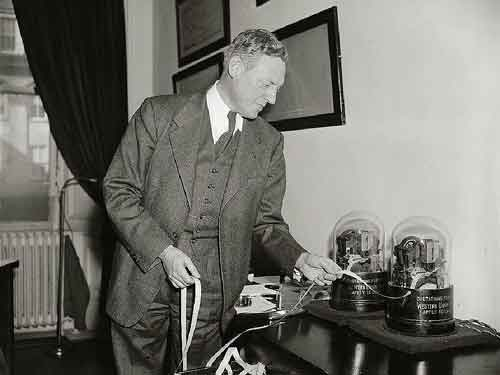ROI is a popular general purpose metric for evaluating investments in stock shares and the use of venture capital, but also for valuing capital purchases, projects, and programs as business investments. [Photo: Economist Archie Lockhead checking share prices and trading action on Western Union tickertape, Washington DC, February, 1939.]ROI is a popular general purpose metric for evaluating investments in stock shares and the use of venture capital, but also for valuing capital purchases, projects, and programs as business investments. [ Photo: Economist Archie Lockhead checking share prices and trading action on Western Union tickertape, Washington DC, February, 1939.]

In fact, businesspeople know several different metrics as "return on investment" or ROI, but usually the term refers to the cash flow metric appearing here as Simple ROI or the Return on Investment Ratio.

This metric compares returns to costs by making a ratio of cash inflows to outflows that follow from the investment. Thus, the ratio is by definition "net investment gains over total investment costs."

• Analysts usually present the ratio as a percentage. When the metric calculates as 0.24, for instance, the analyst reports ROI = 24.0%.
• A positive result such as 24.0% means that returns exceed costs. Analysts, therefore, consider the investment a net gain.
• The opposite kind of outcome, negative results such as –12.7%, mean that costs outweigh returns. Analyst, therefore, view the investment as a net loss.

When comparing two or more investments—and when risks and other factors are equal—analysts prefer the choice with the higher return on investment.

### Return on Investment: Use and Misuse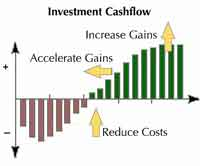Figure 1. Investors and decision-makers use the ROI metric to compare the magnitude and timing of expected gains with the scale and timing of costs.

ROI has become very popular in the last few decades as a general purpose metric for evaluating just about any action or decision that qualifies as a business investment. Remember, however, that many who produce ROI figures have a poor grasp of the metric's weaknesses and unique data needs. With results from an unknown source, therefore, experienced analysts also ask to see the source data for those results.

Some analysts say that "simple" ROI measures profitability. While that statement is accurate and useful, other businesspeople borrow a term from the field of economics and claim that the return on investment metric means efficiency. That usage is arguably less helpful because many people use the same word—"efficiency"—to describe the meaning of quite a few other metrics, including the Internal rate of return IRR, payback period, inventory turns, and return on capital employed (ROCE).

### Explaining Return on Investment ROI in Context

Sections below further define, describe and illustrate return on investment ROI, in context with related terms and concepts from business analysis, investment analysis, and finance.

## Return on Investment Concept Meaning

The name of the return on investment metric describes its meaning. It is not surprising, therefore, that businesspeople use ROI to address questions like these: "What do we receive for what we spend?""Do expected returns outweigh the costs? Or, simply, "Is the action profitable?"

The simple ROI metric answers these questions by making a ratio (or percentage), showing the size of net gains relative to the size of total costs directly.

• When total returns exceed total costs, net gains are positive, and the metric is positive (greater than 0).
• The opposite outcome (negative net gains) leads to a negative metric (less than 0). A negative ROI means, therefore, that total costs outweigh total returns and the investment is a net loss.

A result of 10%, says that returns exceed costs by 10%. And, with a 10% ROI, the investor can rightly say "the action earned 10% on costs" and that "profitability was 10%." In the same way, an result of -10% says that earnings and profitability were negative.

### ROI Does Not Measure Risk

Decision makers should know that return on investment figures alone are not a sufficient basis for choosing one action over another. The problem is that ROI shows how returns compare to costs only if the hoped-for results arrive. The calculated figure, therefore, shows expected profitability but says nothing about uncertainty or risk. Consequently, the wise analyst also estimates the likelihood of different ROI outcomes, and wise decision-makers always consider both the size of the metric and the risks that come with it.

Decision makers will probably expect the analyst to produce ROI figures and also measures of risk, of course, but they will also expect practical advice on ways to improve return on investment by reducing costs, growing income, and moving gains forward in time (as the arrows in the figure above suggest).

## Calculating ROI For Decision Support and Investment Analysis

Analysts usually present return on investment as the return (net gain) due to an action divided by the cost of the act. That is the simple ROI version of the cash flow metric for rating investments, business case results, and other actions.

The analyst will probably receive an ROI request as a question such as this:

What is the return on investment for a marketing program that will cost \$500,000 and deliver an additional \$700,000 in profits over the next five years?

To find simple ROI, divide the net gains from the investment by the investment costs, then report the result as a percentage. Figure 2 shows the these instructions as a formula, along with the marketing program figures that produce a 40.0% result.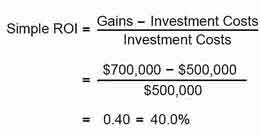Figure 2.The return on investment formula is simple, but usage is not always as simple as it looks. The real challenge in finding ROI for any investment or action is knowing which costs and which return figures belong in the formula.Figure 2.The return on investment formula is simple, but usage is not always as simple as it looks. The real challenge in finding ROI for any investment or action is knowing which costs and which return figures belong in the formula.

Note especially: Results such as the 40.0% figure above mean something only when both the gains and the costs are undoubtedly due to the action, and are not due in part to other causes.

In complicated business settings, however, it is not always easy to match specific returns (such as greater profits) with the specific costs that bring them (such as the costs of a marketing program). As a result, when the match between "returns" and "costs" is doubtful, the ROI metric loses validity as a guide for decision support. ROI validity also suffers when the cost figures include allocations or indirect costs, which are probably not due to the action.

## ROI Compares Compares Cash Flow Streams

Sections immediately below show how ROI metrics compare two investment cases that are competing for funding. Comparisons of this kind turn up when decision-makers must prioritize incoming proposals to choose those that will justify their costs. At the same time, they will deny funding to those that will probably bring smaller returns or even a net loss. As a result, Capital Review Committees, Project Management Officers, strategic planners, and others, routinely turn to metrics that take an "investment view," of proposed actions. When using ROI to compare two proposals, other things being equal, decision-makers will probably choose the option with the higher ROI.

Note especially that other examples below compare these cases again, using ROI along with five other metrics. The purpose of the multi-metric comparison, therefore, is to show that different "metrics" can reach opposite conclusions on which case represents a better business decision. (see "How does return on investment compare to other financial metrics?")

### Example Calculations: Return on Investment for Two Competing Investments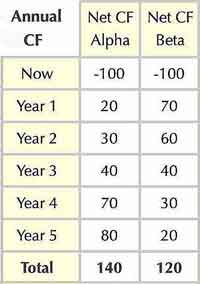Table 1. Five-year net cash flow events for two competing investments.Table 1. Five-year net cash flow events for two competing investments.

Consider two five-year investments competing for funding, Case Alpha and Case Beta. Which is the better choice in business terms? Analysts will probably look first at the expected net cash flow streams for these cases in Table 1.

Notice two features of these cash flow streams that are apparent at once.

• Firstly, Case Alpha has the higher overall net cash flow over five years: Alpha's total net cash flow of \$140 is higher than Beta's \$120.
• Secondly, however, cash flow timing in the two cases is quite different. Beta seems to be "front-loaded," which means that more substantial returns come in the earlier years. As a result, the analyst will want to know how the timing differences impact several different cash flow metrics.

Notice in Figure 3 below that the timing differences stand out clearly, in a net cash flow chart: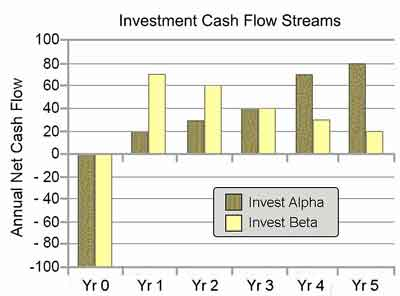Figure 3. Five-year net cash flow events for two competing investments.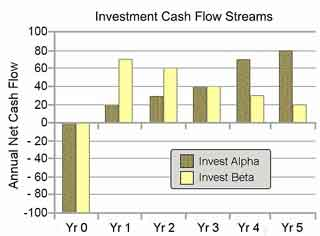Figure 3. Five-year net cash flow events for two competing investments.

### Critical Decisions Deserve MultipleMetrics

As a rule, decision-makers usually consider several financial metrics, not just one, when making important decisions. Consequently, to answer the question, "Which is the better business decision?," the analyst will probably review both cash flow streams with a set of metrics such as ROI, NPV, IRR, and Payback period.

Examples below will show, by the way, that Alpha's cash flow stream has a problem that is hidden by the net cash flow figures. Among these metrics, only ROI reveals this problem. Later sections show that ROI's unique insight has to do with the difference between profits and profitability.

### Data Needs for Calculating Simple Return On Investment

To produce simple ROI, the analyst must have cash inflow and cash outflow data for each period, not just net cash flow values. The data tables above, therefore, must add columns with these figures, as well. Thus, with inflows and outflows now in the first two columns, the tables suffice for producing proper metrics. Decision makers should note especially, however, that metrics built from these numbers are "proper"—have clear meaning—only if the analyst confirms that these cash flows are due to the investment or action, and not to other causes.

### Investment Alpha Cash Flow Data

Table1 above shows net cash flow figures for the 5-year Investment Alpha. Table 2, below, has additional data on the same cash flow events, including each year's cash nflows and outflows, cumulative cash flow, and ROI. Investment Alpha has only one cost event, a cash outflow at the start of investment life (Year 0). Gains begin in Year 1 and increase year by year through.

### Investment Beta Cash Flow Data

Table1 above also shows net cash flow figures for the 5-year Investment Beta. Table 3, below, has additional data on the same cash flow events, including each year's cash nflows and outflows, cumulative cash flow, and ROI. Like Investment Alpha, Beta also has just one cost event, a cash outflow at the start of investment life (Year 0). For Beta, however, gains begin in Year 1 at a high-level but then decrease year by year through Year 5.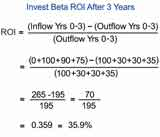Figure 4.The example shows ROI calculations for investment Beta at the end of Year 3.The calculation requires individual inflow and individual outflow figures, not just the annual net figures as in Table 1.Figure 4.The example shows ROI calculations for investment Beta at the end of Year 3.The calculation requires individual inflow and individual outflow figures, not just the annual net figures as in Table 1.

### Which is the Better Business Decision?

Using simple ROI as the sole decision criterion, which choice, Alpha or Beta, is the better business decision? The question equates to asking "Which case has the better returns compared to costs?"

• Comparing 3-year results from each case, Case Beta's 35.9% is greater than Alpha's result of –3.1%. As a result, when other factors are equal, Beta is the better business decision for a 3-year investment.
• Consider 5-year results, Case Beta still has the higher ROI of 51.1%, while Alpha's 5-year figure is less, at 29.5%. Consequently, when other factors are equal, Beta is also the better business decision when the 5-year investment life is in view.

In brief, the return on investment metric shows a significant advantage for Beta, even though Alpha has the greater 5-year net cash flow (\$140 vs. \$120). Why? Note especially that these result from periodic inflows and outflows, not from net cash flows.

Comparing cases

• Alpha has more substantial inflows and outflows.
• Compared to Alpha, Beta's inflows and outflows are smaller.

As a result, Alpha brings in higher profits than Beta, even though Beta is more profitable than Alpha>. Return on investment reveals that difference. NPV, IRR, and Payback metrics are blind to that distinction because they derive from net cash flow figures.

### The Difference Between Profits and Profitability

The differences between profits and profitability can be significant for several reasons, which means, therefore, that some analysts consider an ROI figure mandatory for every investment review. Option Alpha may become less attractive in the investor's eyes, for instance, because he or she must first budget and pay for Alpha's more substantial total costs, no matter how large the incoming returns. As a result, the business decision maker may be unwilling or unable to do so.

## In ConclusionWhen and Where Should You Use ROI?

The use of ROI is usually legitimate when the metric can usefully address questions about investments and decisions such as these:

• Should we make this investment, "Yes" or "No"?
• Does the investment bring a net gain or a net loss?
• What is the profitability of this investment? In other words, what is its return on investment?
• Which of several competing actions is the most profitable? And, which option should we choose?

Also, however, it is important to remember to use return on investment only when the appropriate cash flow data for calculating the metric are available. In brief, this means that ROI is legitimate only when all investment costs (cash outflows) and all returns (cash inflows) are known.

Regarding input data for the metric, therefore, it is helpful to two consider two different situations:

• Return on investment can evaluate straightforward investment situations with only one cash outflow and one cash inflow.
• More often, however, return on investment applies to investment scenarios with multiple cash inflows and outflows across a longer investment life.

### Simple Two-Event ROI Example

For simple action scenarios with only one cash outflow and one cash inflow, ROI data needs are elementary. Here, the analyst needs only two numbers:

• Cash inflow
• Cash outflow

As a simple example, consider this question:

What is the return on investment for a gambler's winning bet on a horse race?

IIn this example, just before the race, the gambler places a \$10 bet on horse #4 to win. Then, a few minutes later, horse #4 finishes first. The pay off for a winning bet depends of course on the "odds" in effect when betting windows close. The resulting ROI, therefore, also depends on the betting odds and the bettor can say, correctly, that these odds are the same as the ROI for this bet. Suppose in this case the winning bet pays \$24. As a result, ROI is 240% for this single investment.

ROI = (24 - 10) / 10 = 240%

There is one cash outflow (the \$10 bet) and one cash inflow (the \$24 pay off). Both events are due to the investment, and the ROI meaning is therefore valid. Note especially that the "two-event " metric result does not take into account the time span between outflow and inflow. Remember also that metric itself is also unconcerned with investment risks or the advisability of making such an investment.

### Using the Two-Event ROI Model

The two-event ROI model applies to other simple investment questions such as these:

• What is the return on investment for ona non-coupon-paying bond purchase?
• What is the return on investment on a work of art, purchased as an investment?

Note that the two-event model assumes there are no necessary owner costs besides the single purchase cost.

### Complex Actions: Multiple Cash Inflows and Outflows over Extended Time Period.

In business, the return on investment metric more often applies to actions that bring many cash flow events across many years. Case Alpha and Case Beta above are examples of the multi-event, multi-year ROI. Here, unlike the simple two-event case, the analyst must, therefore, know the length of investment life. Specifying the lifespan is necessary because this lifespan determines which data go into the ROI ratio.

### Calculating Multi-Year Multi-Period ROI

For the multi-event, multi-year case, therefore, all that the analyst needs the following to calculate return on investment.

• The known length of the investment life
• Total investment costs (cash outflows)
• The sum of returns due to the investment(cash inflows) across investment life

For Case Alpha, the 5-year inflow sum is \$615, and the 5-year outflow sum is \$475. As a result, the Case Alpha metric calculates as 29.5%.

ROI = (615 - 475) / 475 = 29.5%

This result is the "5-year ROI" for Case Alpha. Note that investment time span is relevant because data were available for other time spans as well, such as 3-year metric. Note also, that even though total investment lifespan is important, the result for the entire 5-year life is blind to the timing of inflows and outflows within the investment life. ROI, therefore, contrasts with other cash flow metrics such as NPV, IRR, and Payback Period, which are indeed sensitive to cash flow timing in the investment life. (See the section below, "How does ROI compare to NPV, IRR, Payback, and other financial metrics?")

### Using Multi-Year Multi-Period ROI

In conclusion, multi-year multi-period return on investment figures of this kind can address an extensive range of business questions. For example:

• What is the ROI for a college education?
• Is there a positive ROI for professional training?
• Will the marketing program show a positive return on investment?
• What is the return on investment for buying a restaurant business?

## When and Where to Avoid the ROI Metric

Analysts sometimes provide ROI figures when the ROI metric is not appropriate. Consequently, it is helpful to point out some few everyday situations where the analyst should refrain from using simple ROI.

### Avoid ROI When Cash Flow Figures Are Not Available.

Analysts sometimes approach a cash flow stream starting with "Net cash flow" figures for each period. The Alpha and Beta examples show, however, that net cash flow figures alone do not reveal true profitability (simple ROI) for an investment. To build a proper ROI ratio, therefore, the analyst must uncover the underlying inflows and outflows. Nevertheless, some people, produce ROI figures anyway, using the negative net cash flows as "costs" and the positive net cash flows as "returns." As a result, the meaning of those ROIs is unclear.

### Avoid Simple ROI When Comparing Different Periods.

A four-year ROI for one action does not compare appropriately to a seven-year ROI for another investment. Remember the advice, "Other things being equal, the better choice is the option with the higher ROI." However, when comparing metrics for investments or actions with different time periods, "Other things" are not equal. Consequently, ROIs for different periods do not compare directly.

### Avoid ROI If Cash Flows Have Multiple Causes

This issue becomes important when using ROI to address questions such as these:

• What is the return on investment for a marketing program?

It may be relatively easy to measure costs due exclusively to the program. However, in a situation where gains such as "greater sales" or "increased profits" no doubt result from many actions besides the marketing program, it is therefore difficult to measure the portion of those returns actually due to the program.
• Which business case scenario has the better return on investment?

Business case scenarios typically value future costs and benefits as estimated cash outflows and inflows. When calculating ROI for one or more proposal scenarios, however, the analyst must calculate the metric From incremental cash flow figures. The cash flow increment is the difference between a proposal scenario expected cash flows and a baseline scenario's projected cash flows. "Incremental" values are therefore necessary because these values, alone, measure cost and benefit impacts due only to the action or investment (See the section below, "How is ROI used for evaluating business case scenarios?")

## Calculate ROI From DCF Figures?

Some people in business profer to calculate profitability metrics from discounted cash flow figure, that is, from inflow and outflow present values (PVs). This approach presents few computational problems. When the present values for each inflow and outflow are known, finding the metric itself is as simple as building ROI from non-discounted cash flow figures. Table 5 has the PV data required for the calculation for investment Alpha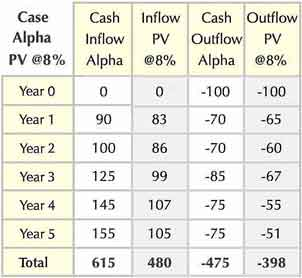Table 5. Data for calculating return on investment from discounted cash flow figures. The calculation rquires present values (PVs) of cash inflows and outflows.Table 5. Data for calculating return on investment from discounted cash flow figures. The calculation rquires present values (PVs) of cash inflows and outflows.

While the mathematics for this calculation are simple, interpreting PV-based metrics can be a challenge. Many businesspeople struggle to properly explain the meaning or use of ROI when the metric results from PV figures. The difficulty interpreting PV-based profitability metrics is no doubt one reason that this approach appears rarely.

For this example, the present value PV for each inflow and outflow represents end-of-period discounting and a discount rate of 8.0% (see Discounted Cash Flow for more on PVs). Under this approach, ROI derives from the sum of the inflow PVs (480) and the total for outflow PVs (398).

Here, the 5-year return on investment for Case Alpha is 21.1%.The same metric is 29.5% for Alpha from non-discounted figures.

Alpha ROIpv = (480 - 398) / 398 = 21.1%

Using similar PV figures for Case Beta above, the sum of Beta inflow PVs is 293, while Beta outflow PVs total 210. As a result, the Case Beta ROI is 39.5%. The same metric is 51.1% when calculated from a non-discounted figure.

Beta ROIpv = (293 - 210) / 398 = 39.5%

### Discount-Based Metrics vs. Non-Discount Metrics

Note, especially, that both PV-based metrics are lower than the same ROIs based on non-discounted cash flow figures. Understanding the reasons for these differences, therefore, is key to understanding what PV-based ROI says about investment cash flow streams.

• The PV-based metric can turn out to be either greater or less than ROI from figures non-discounted data. The reason is that the greater discounting impact can fall either on the cash flow costs (outflows) or the cash flow returns (inflows).
• In cases where more considerable costs come early, and more substantial gains come later, discounting lowers ROI. That is because the discount effect is higher on the late-arriving benefits, while the discounting impact is less for the earlier and more substantial costs.
• For investments or actions where the more significant costs come later, and more substantial gains arrive early, discounting has the opposite effect, that is, discounting raises ROI. That is because, in this case, the discount effect is more significant for the late-arriving costs, while the impact is less for the substantial gains that arrive early.

Alpha and Beta both have "investment curve" profiles because, in both cases, larger costs come early and larger returns come later. As a result, both cases have a PV-based ROI that is less than the ROI from non-discounted cash flows. In such cases, the investor can therefore properly say that ROI becomes more conservative, or more pessimistic when "investment curve" ROIs derive from PV data.

Notice especially, that the size of the discounting impact differs between Alpha and Beta. The discounting effect on Alpha is high while the discounting result on Beta is low. Changing to the PV approach reduces Alpha's ROI by 28.5% (from ROI = 29.5% to ROI = 21.1%), while the same change lowers Beta's ROI by 22.7% (from ROI=51.1 to ROI=39.5%).

This difference is due to the backloaded nature of Alpha's cash flow stream, compared to Beta's frontloaded cash flow stream. Even though both cash flow streams qualify as "investment curves," note that Alpha's more substantial cash inflows arrive later in the 5-year investment life), while Beta's more significant cash flows come earlier in the 5-year life. Alpha's ROI, therefore, suffers a greater discounting impact than Beta. This difference is due to the relatively late arrival of Alpha's substantial returns, which are discounted more heavily than Beta's early gains.,

### PV-Based ROI Conclusions

An earlier Case Alpha example stated that the "ROI result for the entire investment life is blind to the timing of inflows and outflows within the investment life." Note especially, however, that statement does not apply to PV-based ROI because the size of the discounting effect is indeed sensitive to cash flow timing.

To some analysts, the examples above show how the PV based approach adds useful information to the ROI figures, while to others, the same examples show how PV-data confuse the metric's meaning. And, to many businesspeople, the discussion about front loaded and backloaded cash flow streams no doubt seems "theoretical," probably having little practical value for those making real-world investment decisions or for business planning. Therefore, In the interest of clarity and a meaning that is easily understood, many business analysts, investors, and decision-makers decide that bringing PV-based cash flow into the ROI picture "muddies the waters." Consequently, many prefer to avoid discounted data when using ROI, while leaving time-value-of-money concepts to the metrics meant explicitly to handle them: net present value NPV and internal rate of return IRR.

Analysts and decision-makers are free, of course, to use or not use PV-based data for ROI, as they wish. However, those who prefer PV-based ROI's should be sure that everyone involved understands how the ROIs are derived and also how to interpret the discounting effects.

## ROI Results Alongside Other MetricsNet CF, NPV, Payback, IRR and Growth Rate

Decision-makers and investors turn to ROI and other cash flow metrics such as NPV, IRR, and Payback to address questions like these: "What is investment profitability." And, Do the returns justify the costs? And, "What do we get back for what we spend?" In other words, they turn to metrics that take an "investment view" of an action or investment.

Note that several different financial metrics besides ROI serve this purpose. These "Investment View" metrics all compare the timing and sizes of returns and costs. Each investment metric (ROI, IRR, NPV, and Payback Period) has a different way to approach this task and, and as a result, each carries a unique message about the nature of the cash flow stream.

At the same time, however, each of these metrics is blind to particular characteristics of the cash flow streams— features that other financial metrics do see. And, also note, that the different "metrics" can disagree on which of the investments is the better business decision.

All of this leads to these conclusions:

• Just one financial metric should not decide critical decisions.
• When different metrics disagree as to which option is the better choice, decision-makers must examine the current financial situation to decide which to follow..

### Financial Metrics for Alpha and Beta Cash Flow Streams

The different natures of Alpha and Beta results also stand out in the cumulative cash flow graph, below. "Cumulative cash flow" for a period is the sum of all net cash flows through the end of the period, the fourth data column in the tables above. (For more on "cumulative cash flow" and payback, see the articles Cash Flow and Payback Period.) Note especially that some people refer to cash flow graphs such as these as "return on investment curves."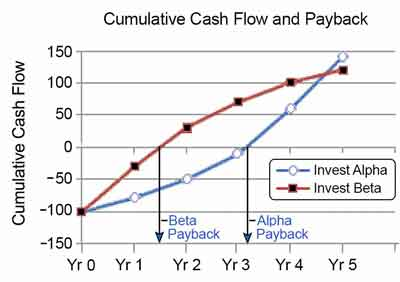Figure 5. Cumulative cash flow curves for the net cash flow figures for Case Alpha and Case Beta. It is not possible to estimate simple ROI from these curves because they represent net cash flow figures, not the cash inflows or cash outflows that make up the net results.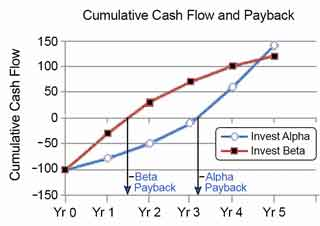Figure 5. Cumulative cash flow curves for the net cash flow figures for Case Alpha and Case Beta. It is not possible to estimate simple ROI from these curves because they represent net cash flow figures, not the cash inflows or cash outflows that make up the net results.

Which case, Alpha or Beta, is the better business decision? The analysis shows that each case has points in its favor, compared to the other, and decision-makers must, therefore, weigh ROI results along with several different metrics to decide which is the best choice for them.

### Five Financial Metrics to Compare with ROI

When comparing different investment choices, here are some metrics to consider:

#### Total Net Cash Flow

When comparing cash flow streams like these, the analyst no doubt turns first of all to the financial metric total net cash flow. For a 5-year period, Alpha's cash flow total is higher at \$140, while Beta's cash flow is less, at \$120. Hence, Case Alpha outscores Beta on the total net cash flow metric. Therefore, the analyst can say that Alpha has higher profits.

#### Future Performance

Future performance is not a financial metric, per se, but while reviewing total net cash flow, an astute analyst will notice that the two cumulative cash flow curves point to very different results for the years after year five. Notice especially that by Year 5, Alpha's cumulative curve is growing at a rapidly increasing rate while Beta's growth seems to be leveling off. If both investments have no impacts after year 5, of course, there will be no "future performance to consider." However, If the analyst believes these investment patterns will continue, Alpha, therefore, scores higher than Beta regarding likely future results.

#### Simple Return on Investment

Among the financial metrics, the analyst will probably turn secondly to the simple ROI figures for each case. Note especially that Beta's 5-year ROI is higher at 51.1% while Alpha's is less, at 29.5%. Therefore, Beta scores higher than Alpha with the ROI metric. As a result, the analyst may choose to report that Beta scores higher in profitability. The analyst may also note that Beta shows greater profitability at every year-end over the five-year period.

#### The Payback Period Metric

The curves above show roughly the point in time when cumulative cash flows "break even," that is when total inflows balance total outflows. This point on the time axis is the payback period for each case. Therefore, payback for Beta is better (i.e., shorter, or less) at 1.5 years, while Alpha's payback is worse (more extended) at 3.14 years. Other things being equal, analysts prefer a shorter payback to a more extended period. Why? The two most important reasons are probably these:

• Analysts prefer the shorter payback period because it means they recover cost expenditures sooner, and these funds are ready for use again, sooner.
• Analysts consider a shorter payback period less risky than a more extended payback period.

Regarding the payback period, therefore, Case Beta scores higher than Case Alpha.

#### The "Net Present Value" NPV Metric

When cash flow returns and costs extend two years or more into the future, almost all analysts will want to compare cash flow streams with the net present value (NPV) metric. Using a 10% discount rate, Beta has a higher NPV of \$76.18, while Alpha's NPV is less, at \$70.51. Consequently, under the time value of money rationale, Case Beta is worth more, today, than Alpha, even though Alpha will return more funds after five years. Case Beta, therefore, outscores case Alpha regarding the NPV metric.

#### The Internal Rate of Return Metric

Finally, in some settings, analysts will compare cash flow streams regarding the internal rate of return metric. The Internal rate of return (IRR) is the interest rate that produces an NPV of 0 for a cash flow stream. In reality, not many people in business are prepared to explain IRR figures in a way that makes practical sense for decision-makers and investors. Nevertheless, financial officers in some industries (such as financial services or insurance) rely on the metric for decision support. (See the article Internal rate of return for more on IRR, problems with IRR usage, and a recommended alternative, modified internal rate of return MIRR).

Here, Case Alpha has the lower IRR rate, at 28.9% while Beta's IRR is higher at 44.9%. Financial officers usually view an investment with an IRR above their cost of capital as a net gain and, here, both cases show IRR well above any company's cost of capital. However, when proposals compete for funds, and when other factors are equal, decision-makers prefer the submission with the higher IRR. In conclusion, case Beta has the advantage regarding IRR.

#### Financial Metrics Comparison Summary

In conclusion, different financial metrics can disagree on which investment is the better business decision. The table below summarizes these differences for this example: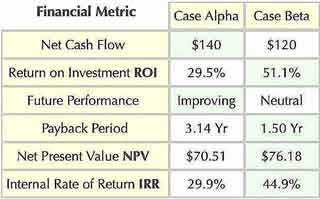Table 6. Summary of financial metrics results comparing investments Alpha and Beta. Green cells hold the better outcome for each financial metric.

From the metrics analysis above, which action, Alpha or Beta, is the better business decision? Four of the metrics in the table favor case Beta while two others favor Case Alpha. In conclusion, there is no "one size fits all" answer, except to say that ROI is a factor that decision-makers and planners will consider. However, they will probably also consider other factors, and then give different weights to each metric. In other words, they will probably appraise such things as:

• The current financial situation
• Risks with each investment
• Other actions that may be available

## Using ROI to Evaluate Business Case Scenarios

Which business case scenario should the analyst recommend? Does the business case justify funding the proposal? ROI and other cash flow metrics (NPV, IRR, and Payback) often play a role in addressing such questions.

The business case looks forward in time, projecting cash inflows (benefits) and cash outflows (costs) under two or more scenarios.

• Firstly, one scenario will represent the proposed action or investment.
• Secondly, however, cases include a "Baseline" or "Business as usual" scenario. Note that the Baseline scenario projects results for the same cost and benefit items without taking the proposed action.

Consequently, the Baseline lets the analyst measure changes (from Baseline) that would follow from implementing the Proposal scenario.

When the analyst has two "full value" scenario cash flow statements (one for the Proposal scenario and another for the Baseline Business as usual), the analyst also builds an incremental cash flow statement. Figures in this statement represent the differences between the same cash flow items in the two full value scenarios, hence the term "incremental." As a result, the incremental cash flow values represent only cash flows due to the action or investment. These values are therefore legitimate data for finding scenario ROIs.

For more on business case cash flow, see Business Case Cash Flow Statement. Also, for ROI examples with business case cash flow, see Business Case Essentials.

## Other Metrics Called Return on Investment ROI?

The return on investment name sometimes applies to several other metrics in financial statement analysis—where analysts assess a company's financial health and earnings results. Some people say ROI when referring to measures also known as:

Average Rate of Return
Return on Total Assets
Return on Equity
Return on Net worth
Earnings per Share
Return on Capital Employed

Also, the term some people refer to cumulative cash flow results over time as a "return on Investment curve." Also, some people call still other cash flow metrics "return on investment," such as the Average Rate of Return and even Internal Rate of Return IRR.

In conclusion, several different "ROI" metrics are in common use. The term itself does not have a single meaning that everyone in business understands in the same way. Therefore, when using return on investment figures, it is good practice to be sure that everyone involved understands precisely which version of the metric is in view.